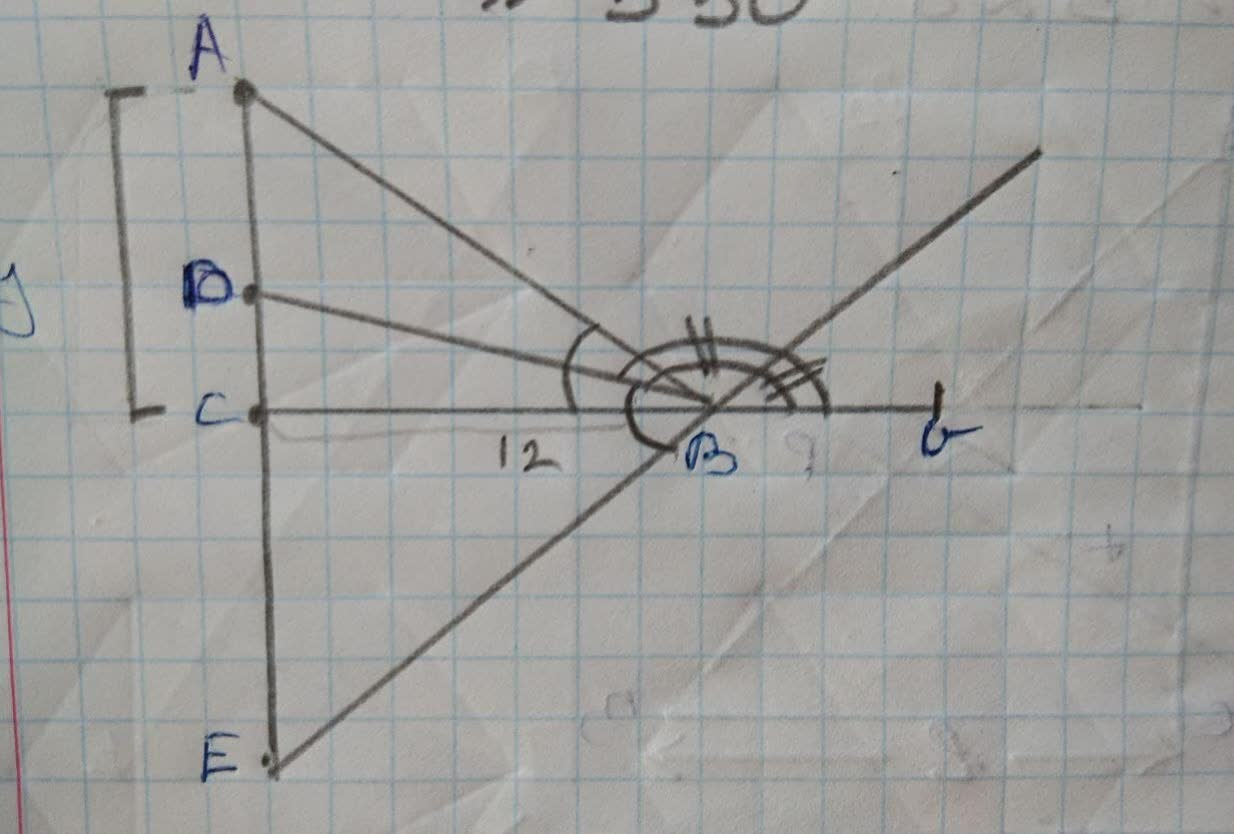triangle ABC is a right triangle with its right angleAshley Searcy 2021-11-27 Answered
triangle ABC is a right triangle with its right angle at C. The bisector of angle B intersects AC at D. The bisector of the exterior angle at B intersects AC at E. If BD= 15 and BE=20, what are the lengths of triangle ABC?

• Questions are typically answered in as fast as 30 minutes

Solve your problem for the price of one coffee

• Math expert for every subject
• Pay only if we can solve itMarian Tucker
Step 1
Using basic properties of triangles we can solve this question.Step2
angle abc is bisected by bd
angle abg is bisected by be
angle dbe=90(half of a angle in a straight line)
bd=15cm
be=20cm
By pythagoras theorem,
de=25cm
Let de=xcm
$$\displaystyle{b}{e}=\sqrt{{{b}{e}^{{{2}}}-{c}{e}^{{{2}}}}}$$
$$\displaystyle=\sqrt{{{b}{d}^{{{2}}}-{d}{c}^{{{2}}}}}$$
$$\displaystyle={12}{c}{m}$$
Step 3
$$\displaystyle\angle{d}{b}{c}={\frac{{{d}{c}}}{{{b}{c}}}}$$
$$\displaystyle={\frac{{{9}}}{{{12}}}}$$
$$\displaystyle{d}{c}={x}$$
$$\displaystyle{e}{c}={25}-{x}$$
$$\displaystyle{b}{e}^{{{2}}}-{c}{e}^{{{2}}}={b}{d}^{{{2}}}-{x}^{{{2}}}$$
$$\displaystyle{x}={d}{c}$$
$$\displaystyle={9}{c}{m}$$
$$\displaystyle\angle{a}{b}{c}={2}\angle{0}{b}{c}$$
$$\displaystyle={\frac{{{24}}}{{{7}}}}$$
$$\displaystyle{\frac{{{24}}}{{{7}}}}={\frac{{{y}}}{{{12}}}}$$
$$\displaystyle{y}={\frac{{{24}\star{12}}}{{{7}}}}$$
$$\displaystyle{a}{c}={\frac{{{288}}}{{{7}}}}$$
By pythagoras theorem
$$\displaystyle{a}{c}^{{{2}}}+{b}{c}^{{{2}}}={a}{b}^{{{2}}}$$
$$\displaystyle{a}{b}={\frac{{{300}}}{{{7}}}}$$